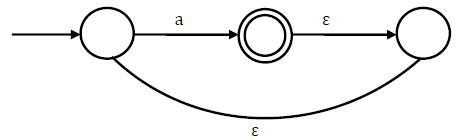Click to Answer GATE Question of the Day

### GATE Question of the Day - Computer Science

1. What is the complement of the language accepted by the NFA shown below? Assume Σ = {a} and is the
empty string(A) ∅
(B) {ε}
(C) a
(D) {a, ε}

2. The protocol data unit (PDU) for the application layer in the Internet stack is

(A) Segment
(B) Datagram
(C) Message
(D) Frame

3. A process executes the code
Fork ();
Fork ();
Fork ();
The total number of child processes created is
(A) 3
(B) 4
(C) 7
(D) 8

4. The decimal value 0.5 in IEEE single precision floating point representation has
(A) Fraction bits of 000…000 and exponent value of 0
(B) Fraction bits of 000…000 and exponents value of -1
(C) Fraction bits of 000…000 and exponents value of -1
(D) No exact representation

5. The worst case running time to search for an element in a balanced in a binary search tree with v2”
elements is
(A) Θ(n log n)
(B) Θ (n2n)
(C) Θ (n)
(D) Θ (log n)

All the Best!!!

saranya said...

1)B 3)C 5)Amuralidharreddy said...

1.b
2.d
3.a
4.d
5.bAnonymous said...

naveen,

1.b
2.c
3.c
4.
5.b